Download Presentation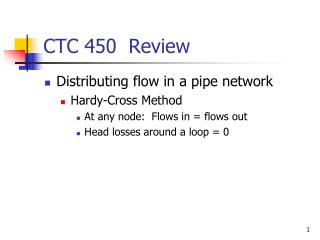CTC 450 Review

# CTC 450 Review - PowerPoint PPT Presentation

CTC 450 Review. Distributing flow in a pipe network Hardy-Cross Method At any node: Flows in = flows out Head losses around a loop = 0. Objectives. Manning’s Equation-Open Channel Flow Rational Method. Uniform Flow in Open Channels.I am the owner, or an agent authorized to act on behalf of the owner, of the copyrighted work described.
Download Presentation## CTC 450 Review

An Image/Link below is provided (as is) to download presentation

Download Policy: Content on the Website is provided to you AS IS for your information and personal use and may not be sold / licensed / shared on other websites without getting consent from its author.While downloading, if for some reason you are not able to download a presentation, the publisher may have deleted the file from their server.

- - - - - - - - - - - - - - - - - - - - - - - - - - E N D - - - - - - - - - - - - - - - - - - - - - - - - - -
Presentation Transcript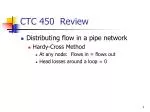CTC 450 Review
• Distributing flow in a pipe network
• Hardy-Cross Method
• At any node: Flows in = flows out
• Head losses around a loop = 0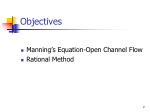Objectives
• Manning’s Equation-Open Channel Flow
• Rational Method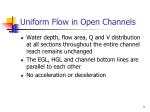Uniform Flow in Open Channels
• Water depth, flow area, Q and V distribution at all sections throughout the entire channel reach remains unchanged
• The EGL, HGL and channel bottom lines are parallel to each other
• No acceleration or decelerationManning’s Equation
• Irish Engineer
• “On the Flow of Water in Open Channels and Pipes” 1891
• Empirical equation
• See more:
• http://manning.sdsu.edu/\
• http://el.erdc.usace.army.mil/elpubs/pdf/sr10.pdf#search=%22manning%20irish%20engineer%22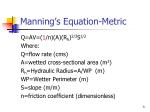Manning’s Equation-Metric

Q=AV=(1/n)(A)(Rh)2/3S1/2

Where:

Q=flow rate (cms)

A=wetted cross-sectional area (m2)

WP=Wetter Perimeter (m)

S=slope (m/m)

n=friction coefficient (dimensionless)Manning’s Equation-English

Q=AV=(1.486/n)(A)(Rh)2/3S1/2

Where:

Q=flow rate (cfs)

A=wetted cross-sectional area (ft2)

WP=Wetter Perimeter (ft)

S=slope (ft/ft)

n=friction coefficient (dimensionless)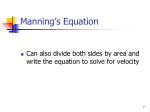Manning’s Equation
• Can also divide both sides by area and write the equation to solve for velocity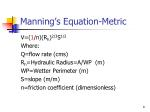Manning’s Equation-Metric

V=(1/n)(Rh)2/3S1/2

Where:

Q=flow rate (cms)

WP=Wetter Perimeter (m)

S=slope (m/m)

n=friction coefficient (dimensionless)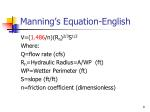Manning’s Equation-English

V=(1.486/n)(Rh)2/3S1/2

Where:

Q=flow rate (cfs)

WP=Wetter Perimeter (ft)

S=slope (ft/ft)

n=friction coefficient (dimensionless)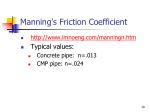Manning’s Friction Coefficient
• http://www.lmnoeng.com/manningn.htm
• Typical values:
• Concrete pipe: n=.013
• CMP pipe: n=.024Example-Find Q

Find the discharge of a rectangular channel 5’ wide w/ a 5% grade, flowing 1’ deep. The channel has a stone and weed bank (n=.035).

A=5 sf; WP=7’; Rh=0.714 ft

S=.05

Q=38 cfsExample-Find S

A 3-m wide rectangular irrigation channel carries a discharge of 25.3 cms @ a uniform depth of 1.2m. Determine the slope of the channel if Manning’s n=.022

A=3.6 sm; WP=5.4m; Rh=0.667m

S=.041=4.1%Friction loss
• How would you use Manning’s equation to estimate friction loss?Triangular/Trapezoidal Channels
• Must use geometry to determine area and wetted perimetersPipe Flow
• Hydraulic radii and wetted perimeters are easy to calculate if the pipe is flowing full or half-full
• If pipe flow is at some other depth, then tables are usually usedUsing Manning’s equation to estimate pipe size
• Size pipe for Q=39 cfs
• Assume full flow
• Assume concrete pipe on a 2% grade
• Put Rh and A in terms of Dia.
• Solve for D=2.15 ft = 25.8”
• Choose a 27” or 30” RCPRational Formula
• Used to estimate peak flows
• Empirical equation
• For drainage areas<200 acres
• Other methods:
• TR-55 (up to 2,000 acres)
• TR-20
• Regression ModelsPeak Runoff Variables
• Drainage area
• Infiltration
• Time of Concentration
• Land Slope
• Rainfall Intensity
• Storage (swamps, ponds)Rational Method
• Q=CIA
• Q is flowrate (cfs)
• C is rational coefficient (dimensionless)
• I is rainfall intensity (in/hr)
• A is drainage area (acres)
• Note: Units work because 1 acre-inch/hr = 1 cfsDerivision
• Assume a storm duration = time of conc.
• Volume of runoff assuming no infiltration

= avg. intensity*drainage area*storm duration

=I*A*TcTheoretical runoff hydrograph

Area under hydrograph = ½ *2Tc*Qp=Tc*QpDerivision of Rational Method
• Volume of rain = Volume observed as Runoff
• I*A*Tc=Tc*Qp
• Qp=IA
• To account for infiltration, evaporation, and storage add a coefficient C (C<1)
• Qp=CIARational Coefficient C
• Don’t confuse w/ Manning’s coefficients
• Typical values:
• Pavement 0.9
• Lawns 0.3
• Forest 0.2

There are also many detailed tables availableRational Coefficient C

Must be weighted if you have different area types within the drainage area

Drainage area = 8 acres:

2 acres; C=0.35 (residential suburban)

6 acres; C=0.2 (undeveloped-unimproved)

Weighted C=[(2)(.35)+(6)(.2)]/8 = 0.24Time of Concentration
• Time required for water to flow from the most distant part of a drainage area to the drainage structure
• Sheet flow
• Shallow, concentrated Flow
• Open Channel FlowIDF Curve
• Shows the relationship between rainfall intensity, storm duration, and storm frequency.
• IDF curves are dependent on the geographical area
• Set time of concentration = storm durationNext Lecture
• Water Quality
• Water Distribution Systems